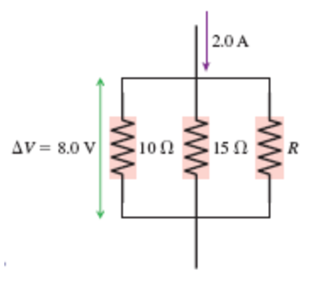# Problem: What is the value of resistor R in the figure below?a. 4.0 Ωb. 12 Ωc. 36 Ωd. 72 Ωe. 96 Ω

###### FREE Expert Solution

From Kirchhoff's junction law, the sum of current into a junction is equal to the sum of current out of the junction.

Current, i = V/R

Current through the first resistor:

i1 = 8/10 = 0.8 A

Current through the second resistor:

i2 = 8/15 = 0.533 A

90% (179 ratings)###### Problem Details

What is the value of resistor R in the figure below?
a. 4.0 Ω
b. 12 Ω
c. 36 Ω
d. 72 Ω
e. 96 Ω